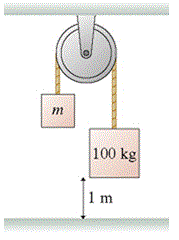# Problem: In the Atwood Machine shown, the 100 kg block in figure takes 5.60 s to reach the floor after being released from rest. (Assume the rope is long enough that the second mass, m, does not hit the pulley before the larger mass reaches the floor, of course.)What is the mass of the block on the left, m?

###### FREE Expert Solution

Use the kinematic relation:

$\overline{){\mathbf{∆}}{\mathbf{h}}{\mathbf{=}}{{\mathbf{v}}}_{{\mathbf{0}}}{\mathbf{t}}{\mathbf{+}}\frac{\mathbf{1}}{\mathbf{2}}{\mathbf{a}}{{\mathbf{t}}}^{{\mathbf{2}}}}$

To get a:

$\begin{array}{rcl}\mathbf{0}\mathbf{-}\mathbf{1}& \mathbf{=}& \mathbf{0}\mathbf{+}\frac{\mathbf{1}}{\mathbf{2}}\mathbf{\left(}\mathbf{a}\mathbf{\right)}{\mathbf{\left(}\mathbf{5}\mathbf{.}\mathbf{60}\mathbf{\right)}}^{\mathbf{2}}\\ \mathbf{a}& \mathbf{=}& \frac{\mathbf{-}\mathbf{2}}{\mathbf{\left(}\mathbf{5}\mathbf{.}\mathbf{60}{\mathbf{\right)}}^{\mathbf{2}}}\end{array}$

a = - 0.0638 m/s2

From Newton's second law:

ΣF = ma

85% (143 ratings)###### Problem Details

In the Atwood Machine shown, the 100 kg block in figure takes 5.60 s to reach the floor after being released from rest. (Assume the rope is long enough that the second mass, m, does not hit the pulley before the larger mass reaches the floor, of course.)What is the mass of the block on the left, m?